# Civil Engineering - Strength of Materials

### Exercise :: Strength of Materials - Section 3

46.

In a simply supported beam L with a triangular load W varying from zero at one end to the maximum value at the other end, the maximum bending moment is

 A.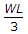B.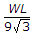C.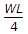D.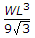E.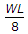Explanation:

No answer description available for this question. Let us discuss.

47.

Maximum deflection of a cantilever due to pure bending moment M at its free end, is

 A.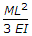B.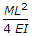C.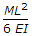D.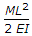E.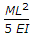Explanation:

No answer description available for this question. Let us discuss.

48.

Along the principal plan subjected to maximum principal stress

 A. maximum shear stress acts B. minimum shear stress acts C. no shear stress acts D. none of these.

Explanation:

No answer description available for this question. Let us discuss.

49.

A diagram which shows the variations of the axial load for all sections of the span of a beam, is called

 A. bending moment diagram B. shear force diagram C. thrust diagram D. stress diagram E. none of these.

Explanation:

No answer description available for this question. Let us discuss.

50.

A member which does not regain its original shape after removed of load producing deformation is said

 A. plastic B. elastic C. rigid D. none of these.